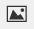PSABPW6Z - Cool-Down---Acc7.5 Lesson 11 More Solutions to Linear Equations (8.EE.C.8.a)
Part A)

A graph of a linear equation passes through (-2,0) and (0,-6).

Use the two points to sketch the graph of the equation.

Draw your graph on paper, take a picture, and upload it using the image upload iconIf you do not have the ability to upload an image of your work, type "Graph is on paper."

Part B)

A graph of a linear equation passes through (-2,0) and (0,-6).

Is 3x y = -6 an equation for this graph?

Select one:
Part C)

A graph of a linear equation passes through (-2,0) and (0,-6).

Is 3x y = -6 an equation for this graph?

Explain how you know.